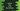# How to do floor division in python## How to do floor division in python:

Floor division is similar to normal division. But it returns the largest number which is less than or equal to the division result.

For example, let’s take a look at the below division:

100/3.

It will give 33 with remainder 1.

Or, we can say that 33 * 3 + 1. The value of 33 is received by using floor division. We can use // to find the floor.

For example, 100//3 will return 25.

### Python example:

Let’s take a look at the below example:

``````print(100//3)
print(300//2)
print(99//10)``````

It will print the below output:

``````33
150
9``````

If I use simple division,

``````print(100/3)
print(300/2)
print(99/10)``````

It will print:

``````33.333333333333336
150.0
9.9``````

## By using math.floor:

There is another method that is called floor defined in math module. We can use this method with any number. So, we can use it with the result of division.

``````import math

print(math.floor(100/3))
print(math.floor(300/2))
print(math.floor(99/10))``````

It will print:

``````33
150
9``````